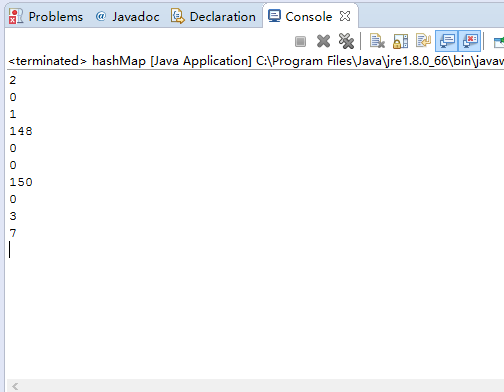# HashMap为什么线程不安全以及解决方法

*注意，此实现不是同步的。如果多个线程同时访问此映射，而其中至少一个线程从结构上修改了该映射，则它必须 保持外部同步。（结构上的修改是指添加或删除一个或多个映射关系的操作；仅改变与实例已经包含的键关联的值不是结构上的修改。）这一般通过对自然封装该映射的对象进行同步操作来完成。如果不存在这样的对象，则应该使用 Collections.synchronizedMap 方法来“包装”该映射。最好在创建时完成这一操作，以防止对映射进行意外的不同步访问，如下所示：
Map m = Collections.synchronizedMap(new HashMap(…));*

hashmap的底层是一个链表散列（数组与链表的结合体）。

1.
void addEntry(int hash, K key, V value, int bucketIndex) { Entry<K,V> e = table[bucketIndex]; table[bucketIndex] = new Entry<K,V>(hash, key, value, e); if (size++ >= threshold) resize(2 * table.length); }
hashmap在调用put方法时会调用上面方法，假若现在同时有A线程和B线程同时获得了同一个头节点并对其进行修改，这样A写入头节点之后B也写入头节点则会将A的操作覆盖

2.

final Entry<K,V> removeEntryForKey(Object key) {
int hash = (key == null) ? 0 : hash(key.hashCode());
int i = indexFor(hash, table.length);
Entry<K,V> prev = table[i];
Entry<K,V> e = prev;

while (e != null) {
Entry<K,V> next = e.next;
Object k;
if (e.hash == hash &&
((k = e.key) == key || (key != null && key.equals(k)))) {
modCount++;
size--;
if (prev == e)
table[i] = next;
else
prev.next = next;
e.recordRemoval(this);
return e;
}
prev = e;
e = next;
}

return e;
}  

3.
void resize(int newCapacity) {
Entry[] oldTable = table;
int oldCapacity = oldTable.length;
if (oldCapacity == MAXIMUM_CAPACITY) {
threshold = Integer.MAX_VALUE;
return;
}

    Entry[] newTable = new Entry[newCapacity];
transfer(newTable);
table = newTable;
threshold = (int)(newCapacity * loadFactor);
}


import java.util.HashMap;
import java.util.Random;
import java.util.concurrent.atomic.AtomicInteger;

public class hashMap {
static void dos(){
HashMap<Long,String> ss=new HashMap<Long,String>();
final AtomicInteger in=new AtomicInteger(0);
ss.put(0L, "ssssssss");
for(int i=0;i<200;i++){
new Thread(new Runnable() {
@Override
public void run() {
// TODO Auto-generated method stub
ss.put(System.nanoTime()+new Random().nextLong(),"ssssssssssss");
String s=ss.get(0L);
if(s==null){
in.incrementAndGet();
}
}
}).start();

}
System.out.println(in);

}
public static void main(String[] args) {
for(int i=0;i<10;i++){
dos();

}

}
}http://flyfoxs.iteye.com/blog/2100120
//两种方式让hashmap线程安全

1.第一种办法api中已经有指出

Map m = Collections.synchronizedMap(new HashMap(…));